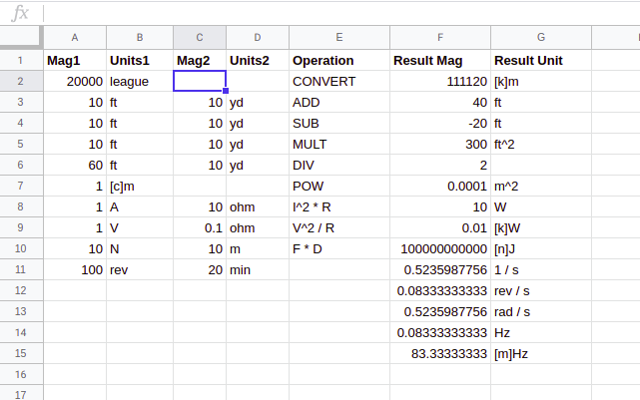Ghost Wrench LLC
•  4Overview
A collection of functions for dealing with numbers with units in Google Sheets. Convert, Add, Subtract, Multiply and More!A collection of functions for dealing with numbers with units in Google Sheets. Convert, Add, Subtract, Multiply and More!
```ConvertPlus extends the built in Google Sheets function "CONVERT" which allows conversion of one quantity (number with a unit of measure) to another unit of measure. ConvertPlus extends this functionality by adding:

* More units
* Explicit pre-fixes that can be added to any unit (e.g. [m]m, [c]m, [k]m)
* Ability to use compond units (e.g. "mi/hr" "k[g] m / s^2")
* Functions for adding, subtracting, multiplying, dividing, raising power of and comparing equality of quantities
* Ability to chain together all functions to avoid multiple cell calculations```
Reviews
Language:
Sort by: